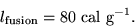# How much ice must melt at 0 deg. C to gain 5 g of mass?

jimmypoopins

## Homework Statement

How much ice must melt at 0 deg. C to gain 5 g of mass?

Latent Heat of Fusion =## Homework Equations

I cannot seem to find many equations that relate thermodynamics and mass, maybe that is my biggest problem... The only one i could find was
Q=Lm

## The Attempt at a Solution

I know the latent heat of fusion, but i do not know Q. I tried dividing both sides by L, giving me Q/L=m, but i wasn't sure where to go from there.